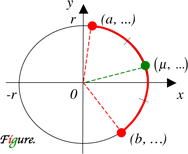Math Central - mathcentral.uregina.caAn Arc Midpoint Computation Proof

Oleksandr (Alex) G. Akulov, student,
University of Waterloo

May 03, 2011Resource Room### Arc Midpoint Computation

If an origin-centered arc of radius $r$ (see Figure) has the ends at $x = a, x = b$ and midpoint at $x = \mu,$ then

$2 \mu = \pm \sqrt{(r + a)(r + b)} \pm \sqrt{(r - a)(r - b)}\; ,$

where the first radical gets “−” iff the arc has a negative x-intercept, and the second radical gets “+” iff  the arc  has a positive x-intercept.

The same relationship holds for y-values.

### Proof

Denote $p=a/r, q=b/r, s_t=(1+t)/2$. Consider angles, $\alpha=\cos^{-1}p, \beta=\cos^{-1}q$, and $\gamma=cos^{-1}(\mu/r)$. There are four cases.

Case 1. The arc does not have $x$-intercepts. Then $\gamma=(\alpha + \beta)/2, \mu=r\cos{1 \over 2}(\cos^{-1}p + \cos^{-1}q)$. Identity $\cos^{-1}p + \cos^{-1}q = 2\cos^{-1}(\sqrt{{s_p}{s_q}} - \sqrt{{s_{-p}}{s_{-q}}})$ gives $\mu = r(\sqrt{{s_p}{s_q}} - \sqrt{{s_{-p}}{s_{-q}}})$. Hence, $2\mu = \sqrt{(r+a)(r+b)} - \sqrt{(r-a)(r-b)}$;Case 2.The arc has positive $x$-intercept, but does not have negative one. Then $\gamma=|\alpha - \beta|/2, \mu=r\cos{1 \over 2}(\cos^{-1}p - \cos^{-1}q)$. Since $|\cos^{-1}p - \cos^{-1}q| = 2\cos^{-1}(\sqrt{{s_p}{s_q}} + \sqrt{{s_{-p}}{s_{-q}}})$, we get $\mu = r(\sqrt{{s_p}{s_q}} + \sqrt{{s_{-p}}{s_{-q}}})$. Hence, $2\mu = \sqrt{(r+a)(r+b)} + \sqrt{(r-a)(r-b)}$;

Case 3. The arc has negative $x$-intercept, but does not have positive one. This part of proof is similar to case 2 with $\gamma = \pi - {1 \over 2}|\alpha - \beta|$;

Case 4. The arc has two $x$-intersepts. This part of the proof is similar to case 1 with $\gamma = \pi - {1 \over 2}(\alpha + \beta)$

Proof for y-values can be received using the same approach or otherwise. Q.E.D.
Arc midpoint computation was suggested by first attempt shown in . Details of proof involved identities received from .

References

1. Oleksandr (Alex) G. Akulov (2009). Dot product finds arc midpoint. Math News, U of W. Volume 111, Issue 6, p. 5.
2. Gregory V. Akulov (2010). The slope of the angle bisector relationship in applied and theoretical problems. Vinculum, SMTS. Volume 2, Number 1, p. 49.about math central :: site map :: links :: notre site français